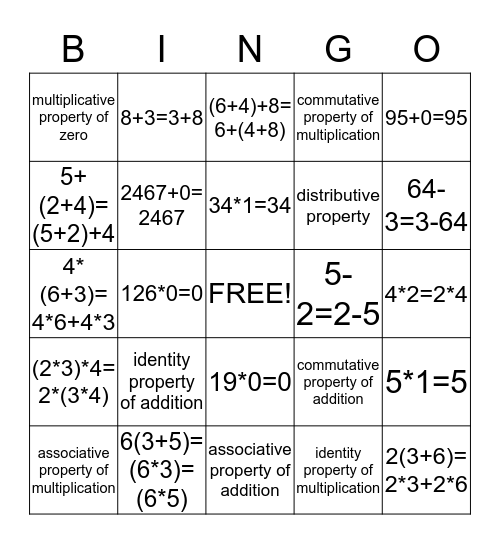# Math Property BingoThis bingo card has a free space and 24 words: identity property of addition, 6(3+5)=(6*3)=(6*5), 34*1=34, associative property of multiplication, 95+0=95, multiplicative property of zero, 5+(2+4)=(5+2)+4, 8+3=3+8, 5-2=2-5, 19*0=0, distributive property, 4*(6+3)= 4*6+4*3, commutative property of addition, 5*1=5, 2467+0= 2467, identity property of multiplication, 4*2=2*4, (6+4)+8= 6+(4+8), 64-3=3-64, 126*0=0, 2(3+6)= 2*3+2*6, (2*3)*4= 2*(3*4), commutative property of multiplication and associative property of addition.

## Play Online

Share this URL with your players:For more control of your online game, create a clone of this card first.

## Probabilities

With players vying for a you'll have to call about __ items before someone wins. There's a __% chance that a lucky player would win after calling __ items.

Tip: If you want your game to last longer (on average), add more unique words/images to it.